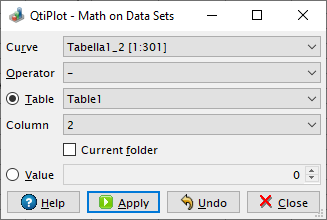# Math on Data Sets Dialog

This dialog is activated by selecting the Subtract -> Reference Data command or the Math on Data Sets... command from the Analysis menu. With its help it is possible to perform basic mathematical operations (additions, substractions, divisions and multiplications) on data curves.

Figure 5-118. The Math on Data Sets dialog.By pressing the Apply button, the arithmetic operation selected in the Operator list box is applied to the current data Curve and to a second operand that can be either an existing data set (a Table column) or a custom Value entered by the user. The last operation can be reverted by pressing the Undo button.

If the second operand is a table column QtiPlot might also use a linear interpolation on the values of this column and the abscissas of the data curve to be modified before performing the requested arithmetic operation.

By checking the Current folder option, only the tables in the active folder of the project are displayed in the Data set list box.